Next: Damped and Driven Harmonic Up: Simple Harmonic Oscillation Previous: Compound Pendula

# Exercises

1. A mass stands on a platform that executes simple harmonic oscillation in a vertical direction at a frequency of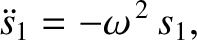. Show that the mass loses contact with the platform when the displacement exceeds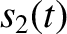. [From Pain 1999.]

2. A small body rests on a horizontal diaphragm of a loudspeaker that is supplied with an alternating current of constant amplitude but variable frequency. If the diaphragm executes simple harmonic oscillation in the vertical direction of amplitude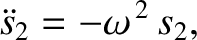, at all frequencies, find the greatest frequency (in hertz) for which the small body stays in contact with the diaphragm.

3. Two light springs have spring constants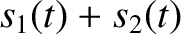and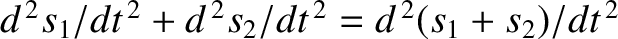, respectively, and are used in a vertical orientation to support an object of mass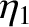. Show that the angular frequency of small amplitude oscillations about the equilibrium state is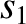if the springs are connected in parallel, and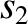if the springs are connected in series.

4. A body of uniform cross-sectional areaand mass density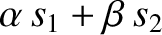floats in a liquid of density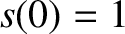(where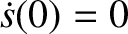), and at equilibrium displaces a volume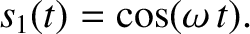. Making use of Archimedes' principle (that the buoyancy force acting on a partially submerged body is equal to the mass of the displaced liquid), show that the period of small amplitude oscillations about the equilibrium position is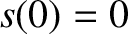5. A particle of massslides in a frictionless semi-circular depression in the ground of radius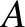. Find the angular frequency of small amplitude oscillations about the particle's equilibrium position, assuming that the oscillations are essentially one-dimensional, so that the particle passes through the lowest point of the depression during each oscillation cycle.

6. If a thin wire is twisted through an angle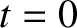then a restoring torque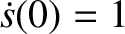develops, whereis known as the torsional force constant. Consider a so-called torsional pendulum, which consists of a horizontal disk of mass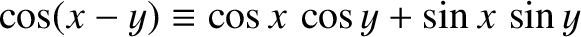, and moment of inertia, suspended at its center from a thin vertical wire of negligible mass and length, whose other end is attached to a fixed support. The disk is free to rotate about a vertical axis passing through the suspension point, but such rotation twists the wire. Find the frequency of torsional oscillations of the disk about its equilibrium position.

7. A circular hoop of diameter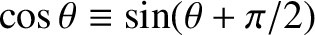hangs on a nail. What is the period of its small amplitude oscillations? [From French 1971.]

8. A compound pendulum consists of a uniform bar of lengththat pivots about one of its ends. Show that the pendulum has the same period of oscillation as a simple pendulum of length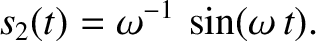.

9. A compound pendulum consists of a uniform circular disk of radius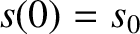that is free to turn about a horizontal axis perpendicular to its plane. Find the position of the axis for which the periodic time is a minimum.

10. A laminar object of masshas a moment of inertiaabout a perpendicular axis passing through its center of mass. Suppose that the object is converted into a compound pendulum by suspending it about a horizontal axis perpendicular to its plane. Show that the minimum effective length of the pendulum occurs when the distance of the suspension point from the center of gravity is equal to the radius of gyration,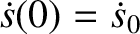.

11. A particle of massexecutes one-dimensional simple harmonic oscillation such that its instantaneous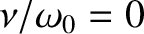coordinate is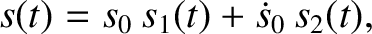Find the average values of,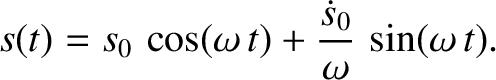,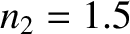, and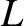over a single cycle of the oscillation. Find the average values of the kinetic and potential energies of the particle over a single cycle of the oscillation.

12. A particle executes two-dimensional simple harmonic oscillation such that its instantaneous coordinates in the-plane are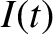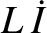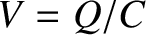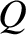Describe the motion when (a)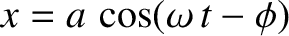, (b)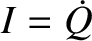, and (c)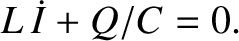. In each case, plot the trajectory of the particle in the-plane.

13. An LC circuit is such that atthe capacitor is uncharged and a currentflows through the inductor. Find an expression for the charge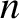stored on the positive plate of the capacitor as a function of time.

14. A simple pendulum of massand lengthis such that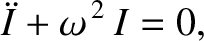and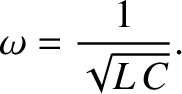. Find the subsequent motion,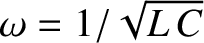, assuming that its amplitude remains small. Suppose, instead, that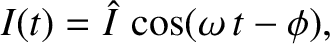and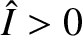. Find the subsequent motion. Suppose, finally, thatand. Find the subsequent motion.

15. Demonstrate that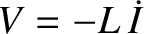is a constant of the motion of a simple pendulum whose time evolution equation is given by Equation (50). (Do not make the small angle approximation.) Hence, show that the amplitude of the motion,, can be written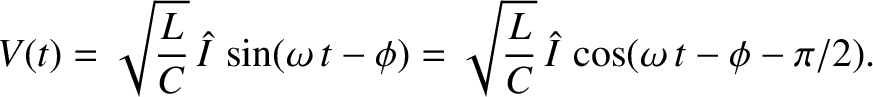Finally, demonstrate that the period of the motion is determined by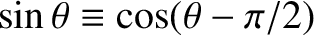where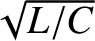is the period of small angle oscillations. Verify that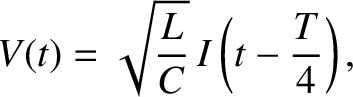as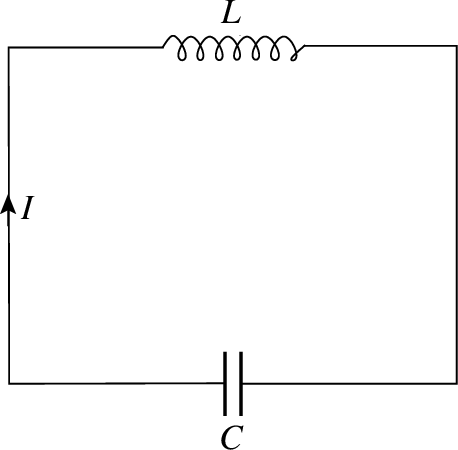. Does the period increase, or decrease, as the amplitude of the motion increases?Next: Damped and Driven Harmonic Up: Simple Harmonic Oscillation Previous: Compound Pendula
Richard Fitzpatrick 2013-04-08